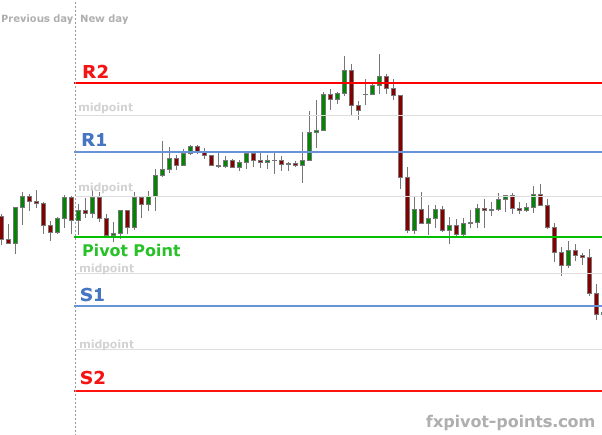Forex Pivot Points Book. Series of Free Forex ebooks

Step-by-step guide and strategy on using Pivot Points in Forex

 HOME | PIVOT POINT CALCULATOR | PIVOT POINTS STRATEGY | WHY PIVOTS WORK | PIVOT TRADING TIPS | CONTACT

 Pages: 1 2 3 4

How to calculate Pivot points?

Pivot levels are derived from previous day High, Low and Close price values. Thus, every new day Pivot points must be reset using the newest data. As a rule traders take the time range from midnight to midnight, e.g. from midnight price bar to midnight bar.

Pivot points study provides traders with 5 major levels:

R2 — Second Resistance
R1 — First Resistance
PP — Pivot Point
S1 — First Support
S2 — Second Support

There are also additional levels, such as R3, S3 — third resistance and support, as well as Mid-points — middle levels between the major levels.There are no limits on how many Pivot levels to use, however, one should remember, that making complex charts makes trading complicated as well. We would suggest sticking to 5 major Pivot point levels, around which most of the price action takes place.

The formula for calculating Pivot points is next:

Major 5 levels:

R2 = Pivot + (High — Low)        (same as R2 = Pivot + (R1 — S1))
R1 = 2 * Pivot — Low
Pivot = (High + Close + Low) / 3
S1 = 2 * Pivot — High
S2 = Pivot — (High — Low)      (same as S2 = Pivot — (R1 — S1))

R3 = High + 2 * (Pivot — Low)
S3 = Low — 2 * (High — Pivot)
Midpoint between R1 and R2 = R1 + (R2 — R1) / 2
Midpoint between Pivot Point and R1 = Pivot + (R1 — Pivot) / 2

Online Pivot Point Calculator

 ► HOME► PIVOT POINT CALCULATOR► PIVOT POINTS STRATEGY► WHY PIVOTS WORK► PIVOT TRADING TIPS► CONTACTFree Forex strategies